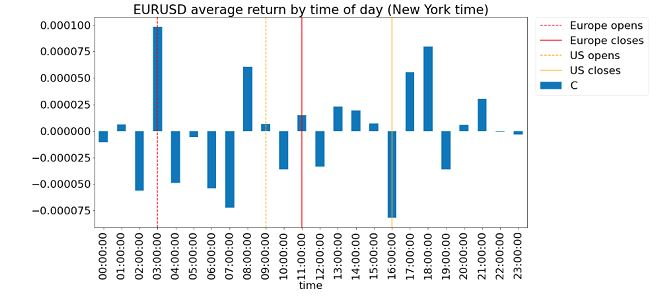## Sabtu, 23 April 2011

### The many facets of linear regression

Many years ago, a portfolio manager asked me in a phone interview: "Do you believe that linear or nonlinear models are more powerful in building trading models?" Being a babe-in-the-woods, I did not hesitate in answering "Nonlinear!" Little did I know that this is the question that separate the men from the boys in the realm of quantitative trading. Subsequent experiences showed me that nonlinear models have mostly been unmitigated disasters in terms of trading profits. As Max Dama said in a recent excellent article on linear regression: "...when the signal to noise ratio is .05:1, ... there’s not much point in worrying about [higher order effects]". One is almost certain to overfit a nonlinear model to non-recurring noise.

Until recently, I have used linear regression mainly in finding hedge ratios between two instruments in pair trading, or more generally in finding the weightings (in number of shares) of individual stocks in a basket in some form of index arbitrage. Of course, others have found linear algebra useful in principal component analysis and more generally factor analysis as well. But thanks to a number of commenters on this blog as well as various private correspondents, I have begun to apply linear regression more directly in trading models.

One way to directly apply linear regression to trading is to use it in place of moving averages. Using moving average implicitly assumes that there is no trend in a price series, that the mean of the prices will remain the same. This of course may not be true. So using linear regression to project the current equilibrium price is sometimes more accurate than just setting it equal to a moving average. I have found that in some cases, this equilibrium price results in better mean-reverting models: e.g. short an instrument when its current price is way above the equilibrium price. Of course, one can also use linear regression in a similar way in momentum models: e.g. if the current price is way above the equilibrium price, consider this a "breakout" and buy the instrument.

Max in his article referenced above also pointed out a more sophisticated version of linear regression, commonly called "weighted least squares regression" (WLS). WLS is to linear regression what exponential moving average (EMA) is to simple moving average (SMA): it gives more weights to recent data points. Indeed I have found that EMA often gives better results than SMA in trading. However, so far I have not found WLS to be better than simple least squares. Max also referenced an article which establishes the equivalence between weighted least squares and Kalman filter. Now Kalman filter is a linear model that is very popular among quantitative traders. The nice feature about Kalman filter is that there is very few free parameters: the model will adapt itself to the means and covariances of the input time series gradually. And furthermore, it can do so one-step at a time (or in technical jargon, using an "online" algorithm) : i.e., there is no need to separate the data into "training" and "test" sets, and no need to define a "lookback" period unlike moving averages. It makes use of "hidden states" much like Hidden Markov Models (HHM), but unlike HHM, Kalman filter is faithfully linear.

I haven't used Kalman filter much myself, but I would welcome any comments from our readers on its usage. Also, if you know of other ways to use linear regression in trading, do share with us here!